BlazingText介绍

Amazon在hogwild、hogbatch之后，提出了《BlazingText: Scaling and Accelerating Word2Vec using Multiple GPUs 》，利用多GPU来加速word2vec的训练。

介绍

word2vec的最优化通过SGD完成，它会进行迭代式求解；在每一个step，会选取一个词对（pair of words）：一个输入词和一个目标词，它们来自于window或一个随机负样本。接着根据选中的两个词来计算目标函数的梯度，然后基于该梯度值更新两个词的词表示（word representations）。该算法接着使用不同的word pair来处理下一次迭代。

SGD的一个主要问题是，它的顺序性；这是因为它在这一轮迭代的更新、与下一轮迭代的计算之间存在着依赖关系（他们可能遇到相同的词表示），每一轮迭代必须潜在等待前一轮迭代的更新完成。这不允许我们使用硬件并行资源。

Hogwild方法在多核架构上的成功，使得该算法利用GPU成为可能，GPU比CPU提供更强的并行化。在该paper中，我们提出了一种有效的并行化技术来使用GPU加速word2vec。

2.word2vec模型

word2vec有两种不同的模型架构：Contextual Bag-Of-Words (CBOW)以及Skip-Gram with Negative Sampling (SGNS) 。CBOW的目标函数是由给定上下文去预测一个词，而Skipgram则给定一个词去预测它的上下文。实际上，Skipgram会给出更好的效果，我们会在下面描述它。

$\sum_{t=1}^T \sum_{c \in C_t} log p(w_c | w_t)$

$p(w_c|w_t) = \frac{exp(s(w_t,w_c))}{\sum_{j=1}^W exp(s(w_t,j))}$

$log(1 + e^{-s(w_t,w_c)}) + \sum_{n \in N_{t,c}} log(1 + e^{s(w_t,n)})$

$\sum_{t=1}^T \sum_{c \in C_t} [l(s(w_t,w_c)) + \sum_{n \in N_{t,c}} l(-s(w_t,n))]$

3.相关工作

Intel的工作展示了通过基于minibatching和shared negative samples的scheme可在多CPU节点上进行极强地扩展。该方法将level-1 BLAS操作转换成level-3 BLAS矩阵乘法操作，从而有效地利用现代架构的向量乘法/加法指令（vectorized multiply-add instructions）。然而，该方法仍不能利用GPUs，而且他们的实现只能在Intel BDW和KNL处理器上扩展良好，而这些处理器比GPU要昂贵，不会被主流的云服务平台所提供。借鉴他们提出的思想，我们可以通过一个minibatch共享negative samples，并使用高度优化的cuBLAS level-3矩阵乘法核（matrix multiplication kernels），但由于要进行乘法操作的矩阵size很小，CUDA核启动（kernel-launches）的开销会剧烈降低性能和可扩展性。

5.实验

5.1 Throughput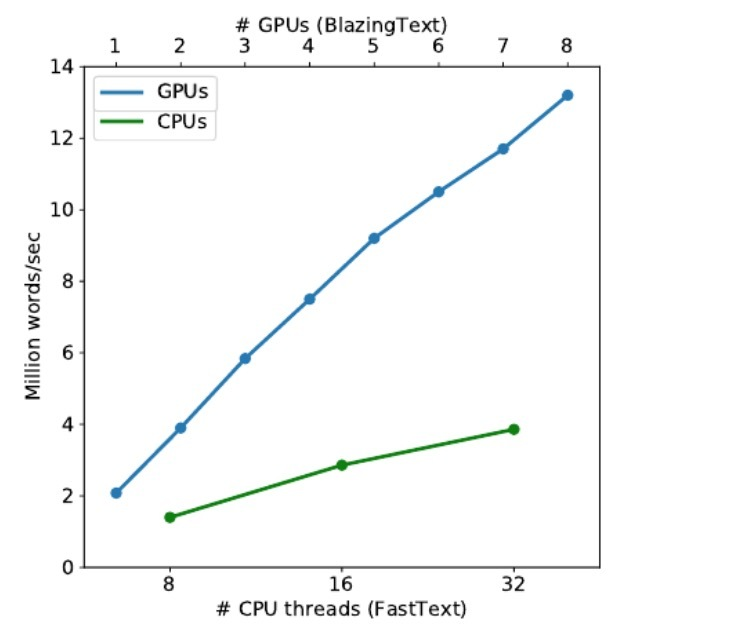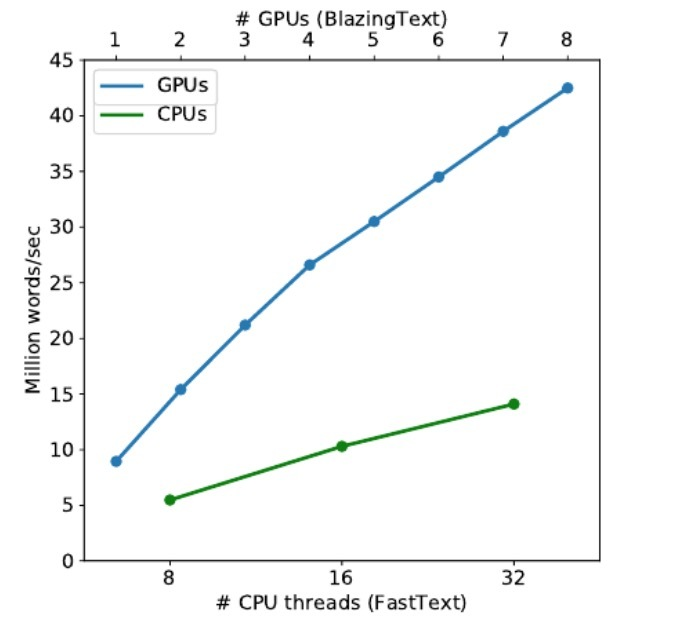5.2 Accuracy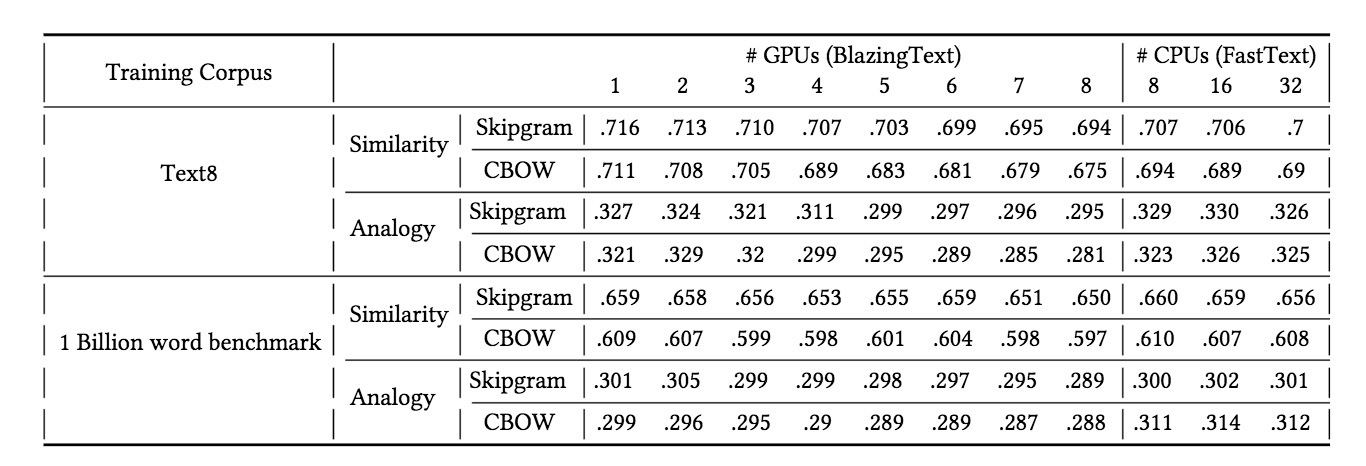3. gcForest算法3.2 Multi-Grained Scanning

DNN在处理特征关系上很强大，例如，CNN在图片数据上很有效（其中原始像素间的空间关系是很重要的）；RNN对于序列型数据很有效（其中序列关系很重要）。受它们的启发，gcForest使用滑动窗口（sliding windows）来扫描原始特征。假设有400个原始特征，我们使用100个特征的window size。对于序列数据，可以通过对每隔一个特征进行窗口滑动来生成一个100维的特征向量；总共可以生成301个特征向量。如果原始特征具有空间关系，比如：在400个图片像素上的20x20的panel，接着一个10x10的window可以产生121个特征向量（比如：121 10x10 panels）。所有的特征向量从正负训练样本上被抽取（忽略正负），接着被用于生成像3.1所述的类向量：从相同size的window中抽取的样本会被用于训练一个completely-random tree forest和一个 random forest，接着生成的类向量被串联作为转换后的特征。如图4所示，假设存在3个类和使用100维的window，对应于一个400维的原始特征向量，会产生一个1806维的转换特征向量。4.实验

4.6 Multi-Grained Scanning的影响参考

https://arxiv.org/pdf/1702.08835.pdf

1.介绍

• 一阶挑战（first-order）：我们展示了一阶神经网络来建模低秩关系的挑战
• Latent Cross: 我们提供了一个简单技术，称为：“Latent Cross”，来在我们的模型上以更有表达力的方式来包含context特征。特别的，latent cross会在context embedding和神经网络的hidden states间执行一个element-wise product。
• 经验结果：我们提供了经验结果来验证在推荐accuracy上的提升。

2.相关工作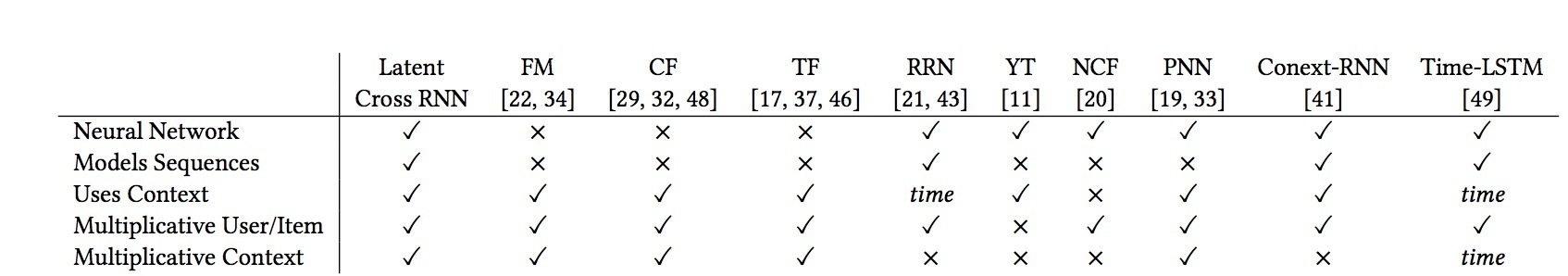3.Preliminaries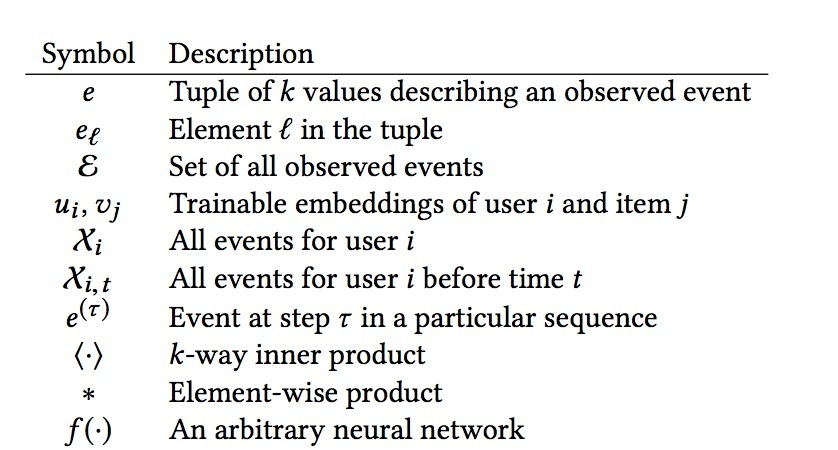4.一阶挑战

$h_{\tau} = g(W_{\tau} h_{(\tau-1)} + b_{\tau})$

…(1)

4.1 建模低秩关系

$u_i \sim \mathcal{N}(0, \frac{1}{r^{(1/2m)}} I)$

…(2)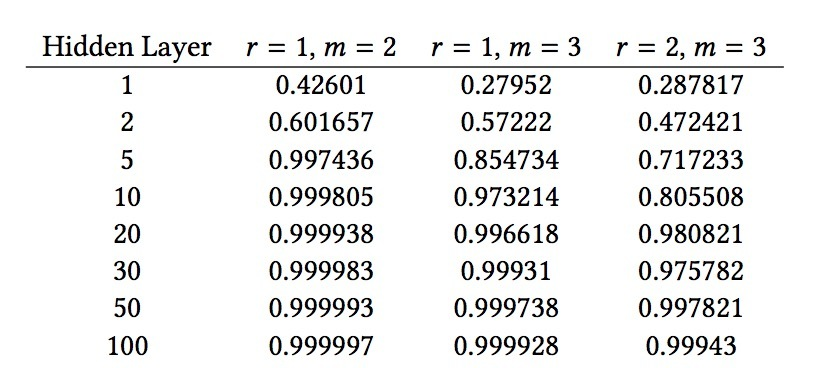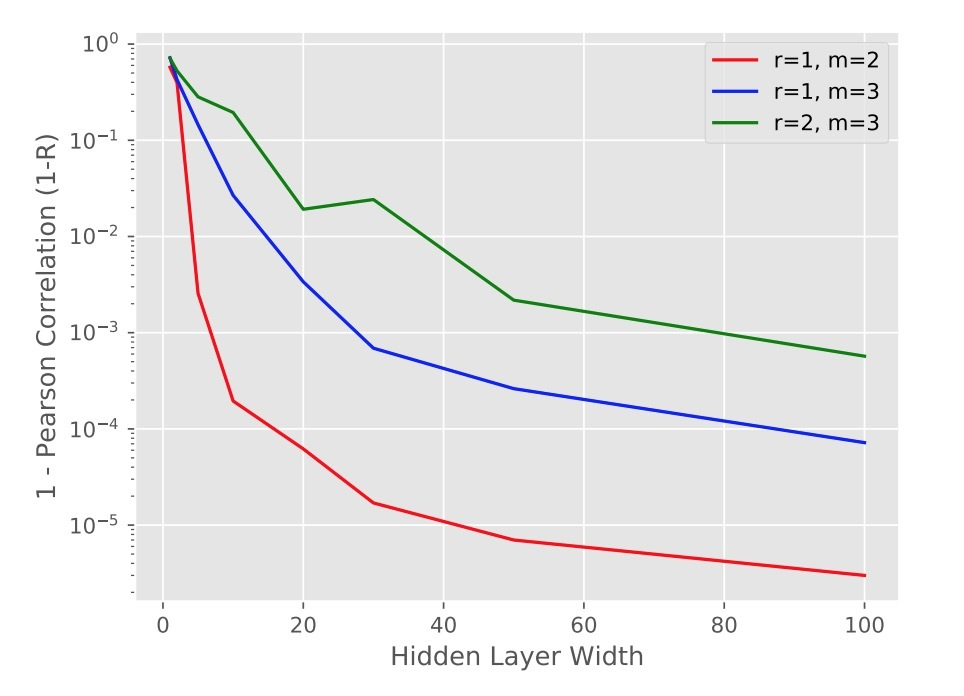5.1 公式描述

• $X_i=\lbrace e=(i,j,\phi(j),t) \in \epsilon \mid e_0 = i \rbrace$：它表示用户的events集合。

$X_{i,t} = \lbrace e = (i,j,t) \in \epsilon | e_0 = i \wedge e_3 < t \rbrace \subset X_i$

…(3)

• $e^{(\tau)}$来表示在序列中的第$\tau$个事件，
• $x^{(\tau)}$用来表示对于$e^{(\tau)}$的转移输入，
• $y^{(\tau)}$表示尝试对第$\tau$个event所预测的label。

• $e^{(\tau)} = (i,j,\phi(j),t)$
• $e^{(\tau+1)} = (i,j',\phi(j'),t')$

• $v_j$是视频embedding
• $u_{\phi(j)}$是上传者embedding
• $w_t$是上下文embedding

5.2 Baseline RNN的结构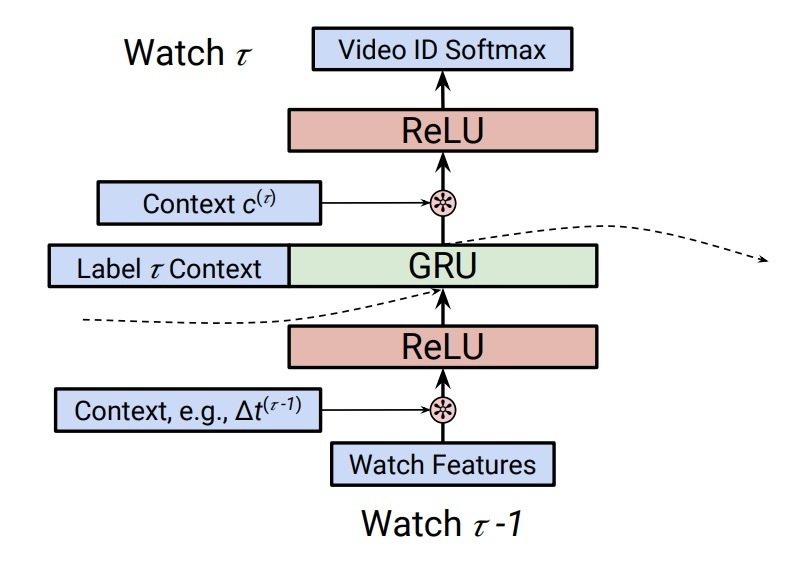5.3 上下文特征

TimeDelta。在我们的系统中，有效对时间进行合并，对于RNN的accuracy很重要。历史上，时间上下文已经以多种方法合并给CF模型中。这里我们使用一种称为timedelta的方法：

$\Delta t^{(\tau)} = log( t^{(\tau+1)} - t^{(\tau)})$

…(4)

Pre-Fusion和Post-Fusion。我们可以使用这些上下文特征，可以称为$c^{(\tau)}$，以两种方式作为直接输入。如图1所示，我们可以将context当成是在该网络底部的一个输入，或者与RNN cell的output进行拼接。我们将在RNN之前的context features包含机制称为“pre-fusion”，在RNN cell之后的context features包含机制称为“post-fusion”。尽管很微妙，该决策对RNN的影响很大。尤其是，通过将pre-fusion中包含一个feature，该feature会在修改RNN的state期间影响预测。然而，通过在post-fusion期间包含一个特征，该特征可以更直接的影响在该step上的预测。

6.使用latent cross进行上下文建模

6.1 单个Feature

$h_0^{(\tau)} = (1+w_t) * h_0^{(\tau)}$

…(5)

$h_1^{(\tau)} = (1+w_t) * h_1^{(\tau)}$

…(6)

6.2 使用多种Features

$h^{(\tau)} = (1+w_t + w_d) * h^{(\tau)}$

…(7)

• (1) 通过使用0均值高斯分布对$w_t$和$w_d$进行初始化，乘法项具有均值为1, 这样可以在hidden state上扮演着mask/attention机制
• (2) 通过一起添加这些项，我们可以捕获在hidden state和每个context feature间的2-way关系。这会遵循FM的设计。
• (3) 使用一个简单的加法函数很容易训练。

7.实验

7.1 比较分析

7.2.2 PV作为context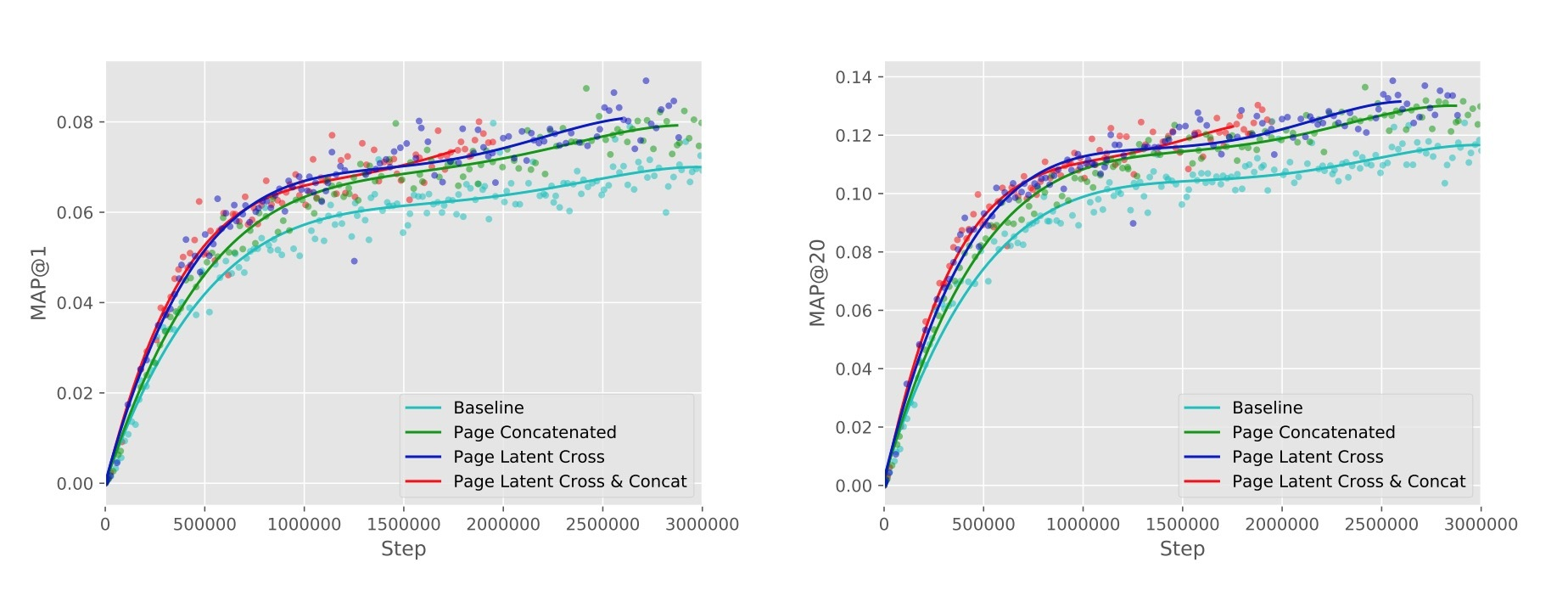7.2.3 总提升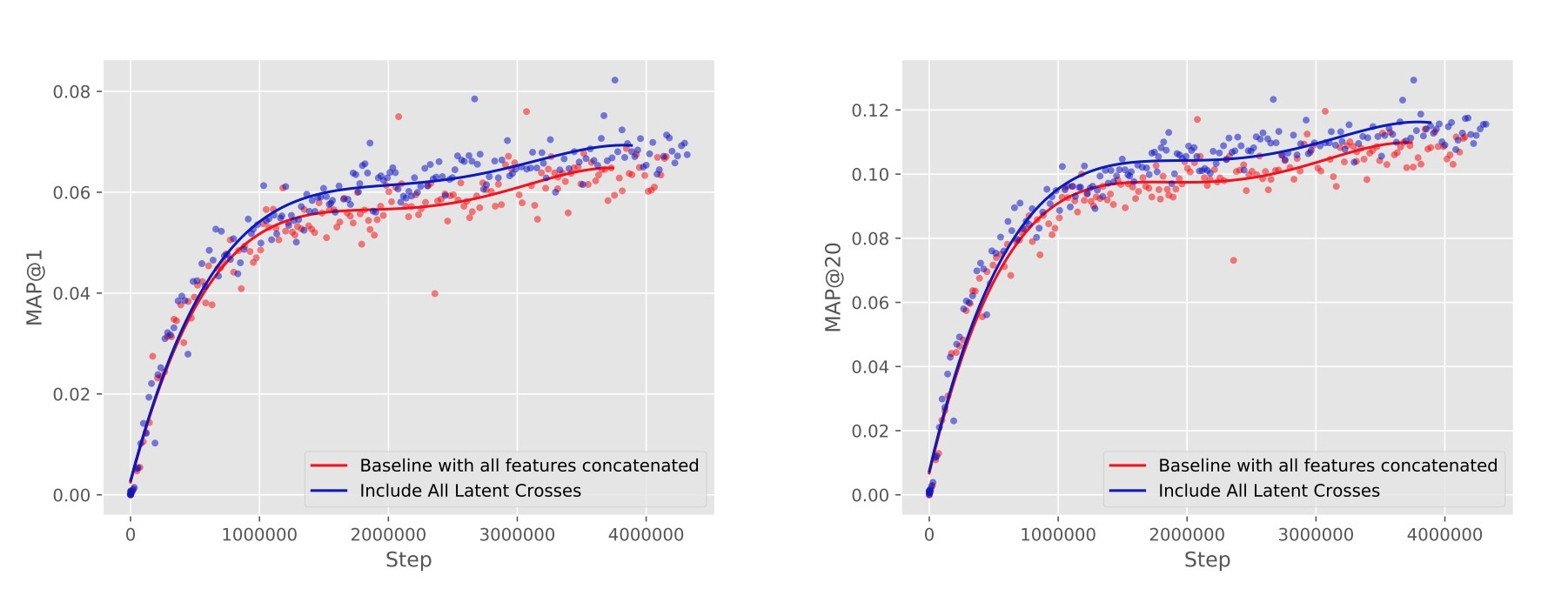8.讨论

8.1 DNN中的离散关系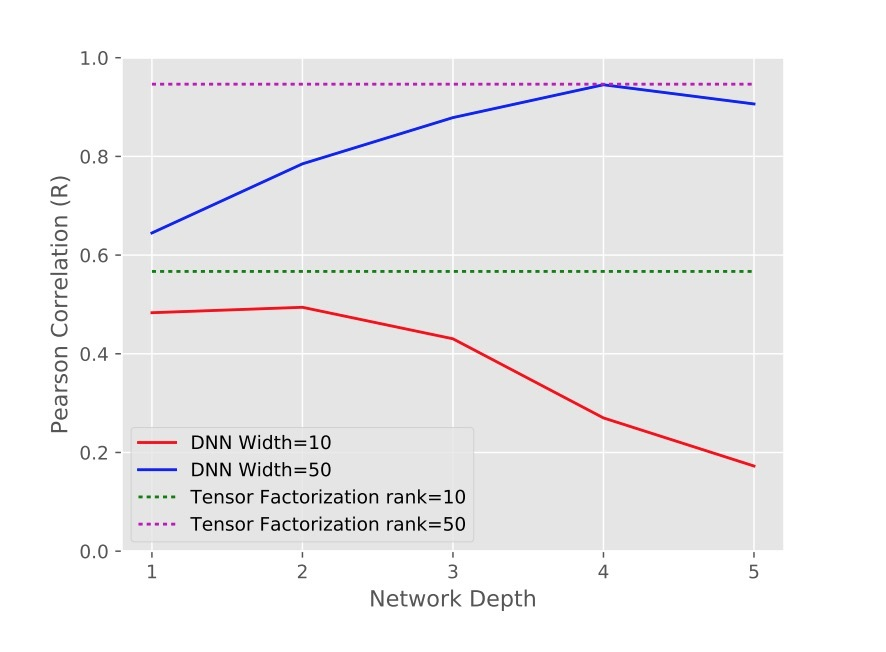1.介绍

• 我们提出了一个新的cross network，它显式地在每个layer上进行特征交叉（feature crossing），可有效学习有限阶（bouned degrees）的特征交叉预测，无需人工特征工程和暴力搜索。
• 该cross network简单有效。通过设计，最高的多项式阶在每一layer递增，由layer depth决定。该网络包含了所有阶的交叉项（直到最高阶），它们的系数都不同。
• 该cross network内存高效，很容易实现。
• 我们的实验结果表明，比起接近相同阶的DNN，DCN具有更低的logloss，更少的参数。

2.DCN2.1 Embedding and Stacking Layer

$x_{embed,i} = W_{embed,i} x_i$

…(1)

$x_0 = [ x_{embed,1}^T, ..., X_{embed,k}^T, X_{dense}^T]$

…(2)

2.2 Cross Network

$x_{l+1} = x_0 x_l^T w_l + b_l + x_l = f(x_l, w_l, b_l) + x_l$

…(3)

• $x_l, x_{l+1}$是列向量（column vectors），分别表示来自第l层和第(l+1)层cross layers的输出；
• $w_l, b_l \in R^d$是第l层layer的weight和bias参数。$d \times L_c \times 2$

cross network的参数数目少，从而限制了模型的能力（capacity）。为了捕获高阶非线性交叉，我们平行引入了一个deep network。

2.3 Deep Network

Deep network是一个fully-connected feed-forward神经网络，每个deep layer具有以下的公式：

$h_{l+1} = f(W_l h_l + b_l)$

…(4)

• $h_l \in R^{n_l}, h_{l+1} \in R^{n_{l+1}}$分别是第l层和第(l+1)层hidden layer；
• $W_l \in R^{n_{l+1} \times n_l}, b_l \in R^{n_{l+1}}$是第l个deep layer的参数；
• $f(\cdot)$是ReLU function。

$d \times m + m + (m^2 + m) \times (L_d - 1)$

2.4 Combination Layer

Combination Layer将两个network的输出进行拼接（concatenate），然后将该拼接向量（concatenated vector）feed 进一个标准的logits layer上。

$p = \sigma ( [x_{L_1}^T, h_{L_2}^T] w_{logits})$

…(5)

• $x_{L_1} \in R^d, h_{L_2} \in R^m$分别是来自cross network和deep network的输出
• $W_{logits} \in R^{d+m}$是combination layer的weight vector，其中$\sigma(x) = 1/(1+exp(-x))$。

loss function是logloss，带有一个正则项。

$loss = - \frac{1}{N} \sum_{i=1}^{N} y_i log(p_i) + (1-y_i) log(1-p_i) + \lambda \sum_{l} \| w_l \|^2$

…(6)

3.Cross Network分析

3.1 多项式近似

$P_n(x)= \{ \sum_{\alpha} w_{\alpha} x_1^{\alpha_1} x_2^{\alpha_2} ... x_d^{\alpha_d} | 0 \le |\alpha| \le n, \alpha \in N^d \}$

…(7)

$\{ \sum_{\alpha} (w_0,...,w_l) x_1^{\alpha_1} x_2^{\alpha_2} ... x_d^{\alpha_d} | 0 \le |\alpha| \le l+1, \alpha \in N^d \}$

• 其中 $c_\alpha = M_\alpha \sum_{i \in B_\alpha} \sum_{j \in P_\alpha} \prod_{k=1}^{|\alpha|} w_{i_k}^{j_k}$, $M_\alpha$是一个与$w_i$独立的常数
• $i = [i_1, ..., i_{|\alpha|}]$和 $j = [j_1, ..., j_{|\alpha|}]$是多元索引（multi-indices）， $B_{\alpha} = \{ y \in \{ 0, 1,...,l\}^{|\alpha |} | y_i < y_j \wedge y_{|\alpha|} = l \}$，
• $P_\alpha$是indice $(\underbrace{1, ..., 1}_{\alpha_1 times} ... \underbrace{d, ..., d}_{\alpha_d times})$的所有排列（permutations）的集合。

3.2 FM的泛化

cross network共享参数，类似于FM模型的参数共享，并扩展到了一个更深的结构上。

FM是一个浅层结构（shallow structure），受限于交叉项的阶是2. 而DCN可以构建所有的交叉项$x_1^{\alpha_1} x_2^{\alpha_2} ... x_d^{\alpha_d}$，其中阶 $|\alpha|$由一些常数决定，见理论3.1。因而，cross network扩展了参数共享的思想，将单个layer扩展到多个layer，并且有更高阶的交叉项。注意，与高阶FM不同的是，在cross network中的参数数目，只随着输入维度线性增长。

3.3 有效投影

$x_p^T = [x_1\tilde{x}_1 ... x_1\tilde{x}_d ... x_d\tilde{x}_1 ... x_d\tilde{x}_d] \left[ \begin{array}{ccc} w&0&...&0\\ 0&w&...&0\\ \vdots&\vdots&\ddots&\vdots\\ 0&0&...&w \end{array} \right]$

…(8)

4.实验结果

Criteo Display Ads数据集用于预测点击率。具有13个integer features和26个categorial features。其中，每个category都具有一个很高的维度基数。对于该数据集，在logloss上提升0.001可以看成是巨大的提升。当考虑到在一个很大的用户基数时，在预测准确率（prediction accuracy）的一个小的提升，可以为公司带到来一个大的回报。数据包含了11GB的包含7天的用户日志（~41000,000条记录）。我们使用前6天的数据做为训练，并将第7天的数据随机划分成等size的validation和test set。

4.2 实现细节

DCN在tensorflow上实现，我们会讨论一些关于训练CDN的细节。

参考

https://arxiv.org/pdf/1708.05123.pdf

2.相关工作

2.1 session-based的推荐

• S是状态集(state)
• A是动作集(action)
• Rwd是一个reward函数
• tr是状态转移函数

3.使用RNN的推荐

RNN可以建模变长序列数据。在RNN和传统前馈深度模型间的主要不同之处是，在构成网络的units中存在一个内部隐状态(hidden state)。标准RNN会更新它们的隐状态h，它使用以下的更新函数：

$h_t = g(W x_t + U h_{t-1})$

…(1)

• g是一个平滑边界函数（比如：使用一个logistic sigmoid函数）
• $x_t$是在时间t上unit的input
• 给定它的当前状态$h_t$, 一个RNN会输出：关于该序列的下一个元素的一个概率分布

GRU是一个关于一个RNN unit的更精巧的模型。它的目标是，解决梯度消失问题(vanishing gradient problem)。GRU gate本质上会学习何时(when)以及多大（how much）进行更新unit的hidden state。GRU的activation是一个在之前activation和候选activation $\hat{h_t}$上的线性插值。

$h_t = (1-z_t) h_{t-1} + z_t \hat{h}_t$

…(2)

$z_t = \sigma(W_z x_t + U_z h_{t-1})$

…(3)

$\hat{h}_t = tanh(W x_t + U(r_t \odot h_{t-1}))$

…(4)

$r_t = \sigma(W_r x_t + U_r h_{t-1})$

…(5)

3.1 定制GRU模型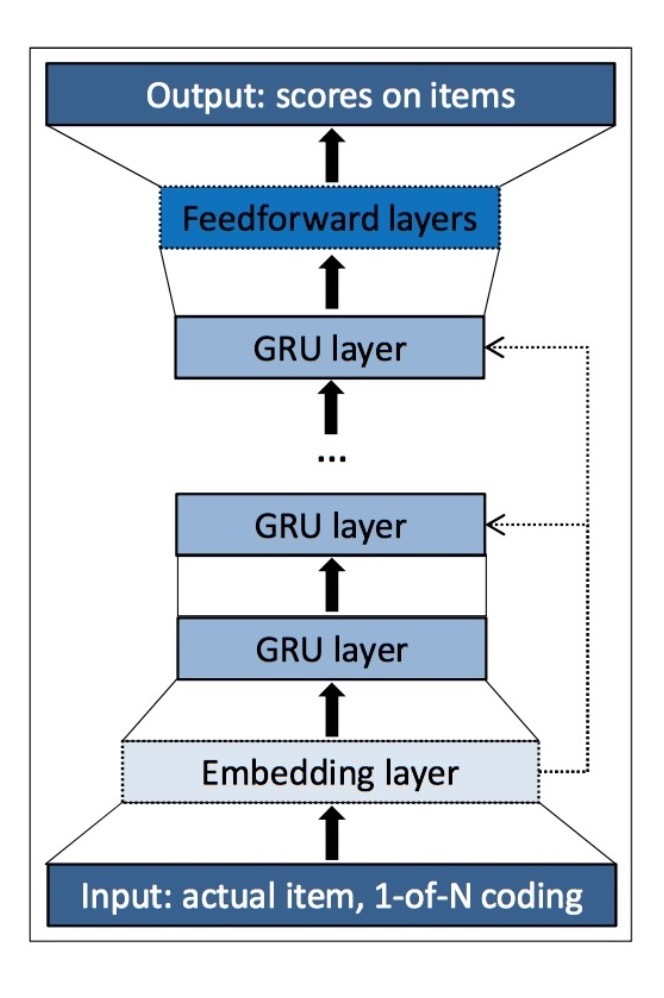3.1.1 session-parallel mini-batches

NLP任务中的RNN通常使用in-sequence mini-batches。例如，很常用的是使用一个slide window来滑过句子中的词，并将这些window化的片段相互挨着来构成mini-batches。这种方法对于我们的任务来说不合适，因为：

• (1) sessions的长度可能非常不同，甚至比句子还要更不同：一些sessions可能只有2个events，而其它可能有上百个；
• (2) 我们的目标是，捕获一个session在时序上的演进，因此将它们分割成片段可能会没有意义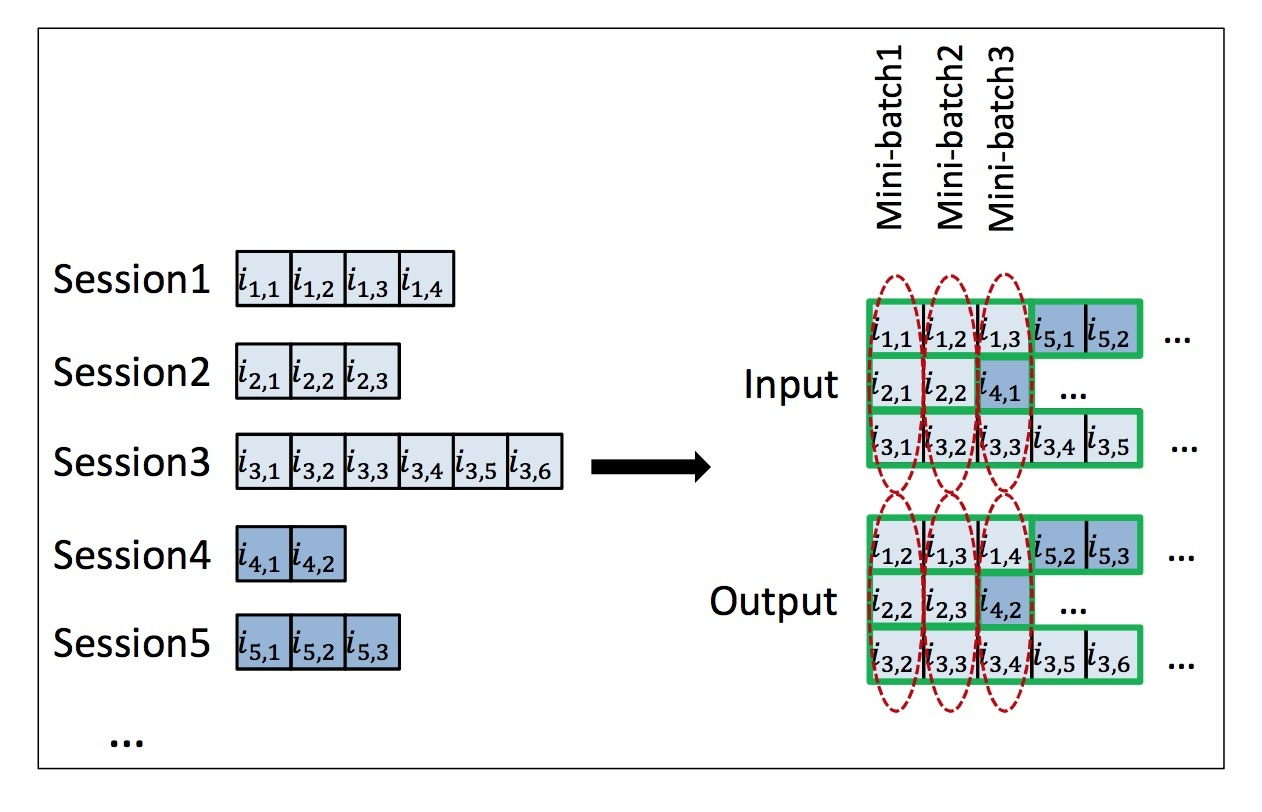3.1.3 ranking loss

• BPR：Bayesian Personalized Ranking (Randle et al., 2009)是一个矩阵因子方法，它使用pairwise ranking loss。它会比较一个positive和一个sampled negative item的score。这里，我们比较了positive item与一些sampled items的scores，并使用它们的平均作为loss。在一个session中对于某个结定点的该loss定义如下：
$L_s = - \frac{1}{N_s} \cdot \sum\limits_{j=1}^{N_s} log(\sigma(\hat{r}_{s,i} - \hat{r}_{s,j}))$

• TOP1: 该ranking loss由我们提出。它是关于相关项的相对rank的正则近似。相关item的相对rank由$\frac{1}{N_s} \cdot \sum\limits_{j=1}^{N_s} I\lbrace \hat{r}_{s,j} > \hat{r}_{s,i} \rbrace$给定。我们使用一个sigmoid来近似$I\lbrace \cdot \rbrace$。这的最优化可以修改参数，以便i的score能高些。然而，这是不稳定的，因为特定positive items也扮演着负样本的角色，因为scores趋向于变得增长更高。为了避免这种情况，我们希望强制负样本的scores在零周围。这是对negative items的scores的一种自然期望。因而，我们添加到一个正则项到该loss中。很重要的是，在相同范围内的该term作为相对rank很重要，。最终的loss function如下所示：
$L_s = \frac{1}{N_s} \cdot \sum\limits_{j=1}^{N_s} \sigma(\hat{r}_{s,j} - \hat{r}_{s,i}) + \sigma(\hat{r}_{s,j}^2)$

4.实验

4.1 Baselines

• POP： 流行度预测，总是推荐训练集中最流行的items。它通常在特定领域内是一个较强的baseline。
• S-POP：该baseline会推荐当前session的最流行items。推荐列表会在session期间随着items获得更多events而进行变化。使用全局流行度值可以突破束缚。该baseline在该领域很强，有较高的重复性。
• Item-KNN：与实际item相似的Item可以通过该baseline被推荐，相似度的定义通过他们sessions向量间的cosine相似度来定义，例如：在sessions中两个items的共现次数，除以各自单个items出现所在的sessions数目的乘积的平方根。可以进行正则化，避免较少访问items的高相似度。该baseline是在实际系统中最常用的item-to-item解决方案，它提供给推荐系统这样的setting：观看了该item的其它人也会观看这些item。尽管它很简单，但它通常是一个较强的baseline。
• BPR-MF：BPR-MF是一个常用的MF方法。它会为一个pairwise ranking目标函数通过SGD进行最优化。矩阵分解不能直接应用到session-based推荐上，因为新的sessions不会具有预计算好的特征向量。然而，我们可以通过使用在session中出现过的item的feature vectors的平均向量来克服这个问题。换句话说，在一个可推荐item和session的items间的特征向量的相似度进行求平均。

参考

https://arxiv.org/pdf/1511.06939.pdf